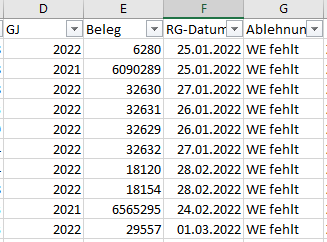# Filter data table by year

Hello,
I need your help again. I have an Excel file that I want to filter by document number “Beleg” and year “GJ”. The file is variable. One time there is only 2022, the next time 2021, 2022 and 2023.

For example, see screenshot. Here I need the output:
DataTable1 = 2021 = 6090289
DataTable2 = 2022 = 6280, 32630, 32631, 32629, 32632Nina

@nina.wenner

I believe you want to filter and get data based on year…

So first read the data using read range into a datatable dt

Then use filter datatable with filter on year with the required year… the output datatable is the required datatable for that particular year…

Repeat this in loop for any numbers of years needed

Cheers

sounds also that you would like to split the datatable into multple datatables each per year

Assign Activity:
TableList | List(Of DataTable) =

``````(From d in YourInputDT.AsEnumerable()
Group d by k=d("GJ").toString.Trim into grp=Group
Select t = grp.CopyToDataTable).toList
``````

ok and how do I get the document numbers column “Beleg” for the current year at the list

Hi @nina.wenner
the easiest way to achieve this solution is using filter datatable activity.
on ‘GJ’ column and save in separate Dt…
conditions may look like - GJ = 2022 and GJ = 2023.
you can also filter not the unnecessary columns from your datatable.

as we have split the input table into different tables we can go to each table and retrieve the Beleg numbers as the same we can process a datatable e.g. for each row.

In case that you are only interested on the list of Beleg numbers we can also creatre a lookup dictionary where key is the year and value a string list with the corresponding beleg numbers

Assign activity:
dictYearBelegLK | Dictionary (Of String, List(Of String)) =

``````(From d in YourInputDT.AsEnumerable()
Group d by k=d("GJ").toString.Trim into grp=Group
Let bl = grp.Select(Function (b) b("Beleg").toString.Trim).toList
Select t = Tuple.Create(k, bl)).toDictionary(Function (x) x.Item1, Function (x) x.Item2)
``````

Also have a look here:

@nina.wenner

Plz check the attached zip file.

cheers
FILTER BY YEAR.zip (8.8 KB)